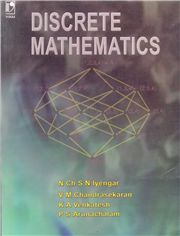# Discrete Mathematics, 1/e N Chsn Iyengar & V M Chandrasekaran-S.CHAND

450.00 405.00

Set Theory
• Matrix Algebra
• Mathematical Logic
• Induction, Recursion and Recurrence Relations
• Algebraic Systems
• Lattices and Boolean Algebra
• Graph Theory
• Formal Languages and Automata